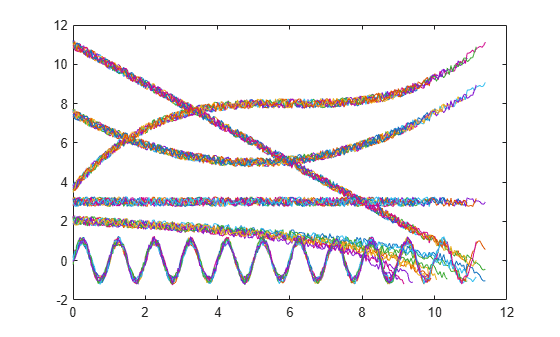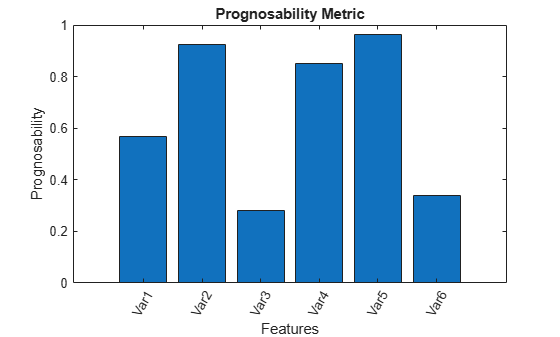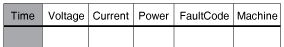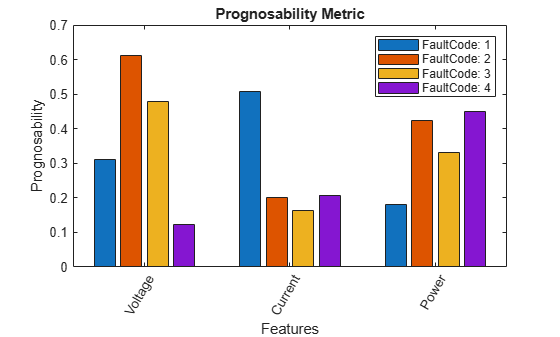# prognosability

Measure of variability of condition indicators at failure

Since R2018b

## Syntax

``Y = prognosability(X)``
``Y = prognosability(X,lifetimeVar)``
``Y = prognosability(X,lifetimeVar,dataVar)``
``Y = prognosability(X,lifetimeVar,dataVar,memberVar)``
``Y = prognosability(___,Name,Value)``
``prognosability(___)``

## Description

example

````Y = prognosability(X)` returns the prognosability of the lifetime data `X`. Use `prognosability` as a measure of the variability of a feature at failure based on the trajectories of the feature measured in several run-to-failure experiments. A more prognosable feature has less variation at failure relative to the range between its initial and final values. The values of `Y` range from 0 to 1, where `Y` is 1 if `X` is perfectly prognosable and 0 if `X` is non-prognosable.```

example

````Y = prognosability(X,lifetimeVar)` returns the prognosability of the lifetime data `X` using the lifetime variable `lifetimeVar`.```

example

````Y = prognosability(X,lifetimeVar,dataVar)` returns the prognosability of the lifetime data `X` using the data variables specified by `dataVar`.```

example

````Y = prognosability(X,lifetimeVar,dataVar,memberVar)` returns the prognosability of the lifetime data `X` using the lifetime variable `lifetimeVar`, the data variables specified by `dataVar`, and the member variable `memberVar`.```

example

````Y = prognosability(___,Name,Value)` estimates the prognosability with additional options specified by one or more `Name,Value` pair arguments. You can use this syntax with any of the previous input-argument combinations.```

example

````prognosability(___)` with no output arguments plots a bar chart of ranked prognosability values.```

## Examples

collapse all

In this example, consider the lifetime data of 10 identical machines with the following 6 potential condition indicators$-$constant, linear, quadratic, cubic, logarithmic, and periodic. The data set `machineDataCellArray.mat` contains `C` which is a `1x10` cell array of matrices where each element of the cell array is a matrix that contains the lifetime data of a machine. For each matrix in the cell array, the first column contains the time while the other columns contain the data variables.

```load('machineDataCellArray.mat','C') display(C)```
```C=1×10 cell array {219x7 double} {189x7 double} {202x7 double} {199x7 double} {229x7 double} {184x7 double} {224x7 double} {208x7 double} {181x7 double} {197x7 double} ```
```for k = 1:length(C) plot(C{k}(:,1), C{k}(:,2:end)); hold on; end```Observe the 6 different condition indicators–constant, linear, quadratic, cubic, logarithmic, and periodic–for all 10 machines on the plot.

Visualize the prognosability of the potential condition indicators.

`prognosability(C)`From the histogram plot, observe that the features `Var2`, `Var4` and `Var5 `rank better than the others. Hence, these features are more appropriate for remaining useful life predictions since they are the best indicators of machine health.

In this example, consider the lifetime data of 10 identical machines with the following 6 potential condition indicators$-$constant, linear, quadratic, cubic, logarithmic, and periodic. The data set `machineDataTable.mat` contains `T`, which is a `1x10` cell array of tables where each element of the cell array contains a table of lifetime data for a machine.

```load('machineDataTable.mat','T'); display(T)```
```T=1×10 cell array {219x7 table} {189x7 table} {202x7 table} {199x7 table} {229x7 table} {184x7 table} {224x7 table} {208x7 table} {181x7 table} {197x7 table} ```
`head(T{1},2)`
``` Time Constant Linear Quadratic Cubic Logarithmic Periodic ____ ________ ______ _________ ______ ___________ ________ 0 3.2029 11.203 7.7029 3.8829 2.2517 0.2029 0.05 2.8135 10.763 7.2637 3.6006 1.8579 0.12251 ```

Note that every table in the cell array contains the lifetime variable `'Time'` and the data variables `'Constant'`, `'Linear'`, `'Quadratic'`, `'Cubic'`, `'Logarithmic'`, and `'Periodic'`.

Compute prognosability with `Time` as the lifetime variable.

`Y = prognosability(T,'Time')`
```Y=1×6 table Constant Linear Quadratic Cubic Logarithmic Periodic ________ _______ _________ _______ ___________ ________ 0.56697 0.92321 0.28044 0.85048 0.96475 0.33853 ```

From the resultant table of prognosability values, observe that the linear, cubic, and logarithmic features have values closer to 1. Hence, these three features are more appropriate for predicting remaining useful life since they are the best indicators of machine health.

Consider the lifetime data of 4 machines. Each machine has 4 fault codes for the potential condition indicators$-$voltage, current, and power. `prognosabilityEnsemble.zip` is a collection of 4 files where every file contains a timetable of lifetime data for each machine - `tbl1.mat`, `tbl2.mat`, `tbl3.mat` and `tbl4.mat`. You can also use files containing data for multiple machines. For each timetable, the organization of the data is as follows:When you perform calculations on tall arrays, MATLAB® uses either a parallel pool (default if you have Parallel Computing Toolbox™) or the local MATLAB session. To run the example using the local MATLAB session, change the global execution environment by using the `mapreducer` function.

`mapreducer(0)`

Extract the compressed files, read the data in the timetables, and create a `fileEnsembleDatastore` object using the timetable data. For more information on creating a file ensemble datastore, see `fileEnsembleDatastore`.

```unzip prognosabilityEnsemble.zip; ens = fileEnsembleDatastore(pwd,'.mat'); ens.DataVariables = {'Voltage','Current','Power','FaultCode','Machine'}; ens.ReadFcn = @readtable_data; ens.SelectedVariables = {'Voltage','Current','Power','FaultCode','Machine'};```

Visualize the prognosability of the potential condition indicators with `'Machine'` as the member variable and group the lifetime data by `'FaultCode'`. Grouping the lifetime data ensures that `prognosability` calculates the metric for each fault code separately.

`prognosability(ens,'MemberVariable','Machine','GroupBy','FaultCode');`
```Evaluating tall expression using the Local MATLAB Session: - Pass 1 of 1: Completed in 0.14 sec Evaluation completed in 0.3 sec Evaluating tall expression using the Local MATLAB Session: - Pass 1 of 1: Completed in 0.039 sec Evaluation completed in 0.16 sec Evaluating tall expression using the Local MATLAB Session: - Pass 1 of 1: Completed in 0.2 sec Evaluation completed in 0.23 sec ````prognosability` returns a histogram plot with the features ranked by their prognosability values. A higher prognosability value indicates a more suitable condition indicator. For instance, the candidate feature `Current` has the highest degree of prognosability for machines with `FaultCode` `1`.

## Input Arguments

collapse all

Lifetime data, specified as a cell array of matrices, cell array of tables and timetables, `fileEnsembleDatastore` object, table, or timetable. Lifetime data contains run-to-failure data of the systems being monitored. The term lifetime here refers to the life of the machine defined in terms of the units you use to measure system life. Units of lifetime can be quantities such as the distance traveled (miles), fuel consumed (gallons), or time since the start of operation (days).

If `X` is

• a cell array of matrices or tables, the function assumes that each matrix or table contains columns of lifetime data for a system. Each column of every matrix or table, except the first column, contains data for a prognostic variable. `'Var1','Var2', ...` can be used to refer to the matrix columns that contain the lifetime data. For instance, the file `machineDataCellArray.mat` contains a 1-by-10 cell array of matrices `C`, where each of the 10 matrices contains data for a particular machine.

• a table or timetable, the function assumes that each column, except the first one, contains columns of lifetime data. The table variable names can be used to refer to the columns that contain the lifetime data. If `lifetimeVar` is not specified when `X` is a table, then the first data column is used as the lifetime variable.

• a `fileEnsembleDatastore` object, specify the data variables `dataVar` and member variables `memberVar` to be used. If `lifetimeVar` is not specified, then the first data column is used as the lifetime variable for computation.

Each numerical member in `X` is of type `double`.

Lifetime variable, specified as a string or character vector. `lifetimeVar` measures the lifetime of the systems being monitored and the lifetime data is sorted with respect to `lifetimeVar`. The value of `lifetimeVar` must be a valid ensemble or table variable name.

For a cell array of matrices, the value `'Time'` can be used to refer to the first column of each matrix, which is assumed to contain the lifetime variable. For instance, the file `machineDataCellArray.mat` contains the cell array `C`, where the first column in each matrix contains the lifetime variable while the other columns contain the data variables.

Data variables, specified as a string array, character vector, or cell array of character vectors. Data variables are the main content of the members of an ensemble. Data variables can include measured data or derived data for the analysis and development of predictive maintenance algorithms.

If `X` is

• a `fileEnsembleDatastore` object, the value of `dataVar` supersedes the `DataVariables` property of the ensemble.

• a cell array of matrices, the value `'Time'` can be used to refer to the first column of each matrix, that is, the lifetime variable `lifetimeVar`. `'Var1','Var2', ...` can be used to refer to the other matrix columns which contain the lifetime data. For instance, the file `machineDataCellArray.mat` contains the cell array `C` where the first column in each matrix contains the lifetime variable. The other columns in the cell array `C` contain the data variables.

• a table, the table variable names can be used to refer to the columns which contain the lifetime data.

The values of `dataVar` must be valid ensemble or table variable names. If `dataVar` is not specified, the computation includes all data columns except the one specified in `lifetimeVar`. For instance, suppose that each entry in a cell array is a table with variables `A`, `B`, `C`, and `D`. Setting `dataVar` to `["A","D"]` uses only `A` and `D` for the computation while `C` and `D` are ignored.

Member variable, specified as a string or character vector. Use `memberVar` to specify the variable for identifying the systems or machines in lifetime data `X`. For instance, in the `fileEnsembleDatastore` object, the fifth column in each timetable contains numbers that identify data from a particular machine. The column name corresponds to the member variable `memberVar`.

`memberVar` is ignored when `X` is specified as a cell array of matrices or tables.

### Name-Value Arguments

Specify optional pairs of arguments as `Name1=Value1,...,NameN=ValueN`, where `Name` is the argument name and `Value` is the corresponding value. Name-value arguments must appear after other arguments, but the order of the pairs does not matter.

Before R2021a, use commas to separate each name and value, and enclose `Name` in quotes.

Example: `...,'WindowSize',0`

Lifetime variable, specified as the comma-separated pair consisting of '`LifeTimeVariable`' and either a string or character vector. If '`LifeTimeVariable`' is not specified, then the first data column is used.

'`LifeTimeVariable`' is equivalent to the input argument `lifetimeVar`.

Data variables, specified as the comma-separated pair consisting of '`DataVariables`' and either a string array, character vector or cell array of character vectors.

'`DataVariables`' is equivalent to the input argument `dataVar`.

Member variables, specified as the comma-separated pair consisting of '`MemberVariable`' and either a string or character vector.

'`MemberVariable`' is equivalent to the input argument `memberVar`.

Grouping criterion, specified as the comma-separated pair consisting of '`GroupBy`' and either a string or character vector. Use '`GroupBy`' to specify the variables for grouping the lifetime data `X` by operating conditions.

The function computes the metric separately for each group that results from applying the criterion, such as a fault condition, specified by '`GroupBy`'. For instance, in the `fileEnsembleDatastore` object `ens`, the fourth column in each timetable in `ens` contains the variable `'FaultCode'`. The metric is computed for each machine by grouping the data by `'FaultCode'`.

You can only group variables when `X` is defined as a `fileEnsembleDatastore` object, table, timetable, or cell array of tables or timetables.

## Output Arguments

collapse all

Prognosability of lifetime data, returned as a vector or table.

Prognosability is the measure of the variability of a feature at failure based on the trajectories of the feature measured in several run-to-failure experiments. A more prognosable feature has less variation at failure relative to the range between its initial and final values. As a system gets progressively closer to failure, a suitable condition indicator is typically highly prognosable. Conversely, any feature that is non-prognosable is a less suitable condition indicator. The values of `Y` range from 0 to 1.

• `Y` is 1 if `X` is perfectly prognosable.

• `Y` is 0 if `X` is perfectly non-prognosable.

Selecting appropriate estimation parameters out of all available features is the first step in building a reliable remaining useful life prediction engine. The prognosability values in `Y` are useful to determine which condition indicators best track the degradation process of systems being monitored. The higher the prognosability, the more desirable the feature is for RUL prediction.

When '`GroupBy`' is not specified, then `Y` is returned as a row vector or single-row table. Conversely, when '`GroupBy`' is specified, then each row in `Y` corresponds to one group.

## Limitations

• When `X` is a tall table or tall timetable, `prognosability` nevertheless loads the complete array into memory using `gather`. If the memory available is inadequate, then `prognosability` returns an error.

## Algorithms

The computation of prognosability uses this formula:

where xj represents the vector of measurements of a feature on the jth system, variable M is the number of systems monitored, and Nj is the number of measurements on the jth system.

 Coble, J., and J. W. Hines. "Identifying Optimal Prognostic Parameters from Data: A Genetic Algorithms Approach." In Proceedings of the Annual Conference of the Prognostics and Health Management Society. 2009.

 Coble, J. "Merging Data Sources to Predict Remaining Useful Life - An Automated Method to Identify Prognostics Parameters." Ph.D. Thesis. University of Tennessee, Knoxville, TN, 2010.

 Lei, Y. Intelligent Fault Diagnosis and Remaining Useful Life Prediction of Rotating Machinery. Xi'an, China: Xi'an Jiaotong University Press, 2017.

 Lofti, S., J. B. Ali, E. Bechhoefer, and M. Benbouzid. "Wind turbine high-speed shaft bearings health prognosis through a spectral Kurtosis-derived indices and SVR." Applied Acoustics Vol. 120, 2017, pp. 1-8.